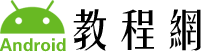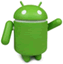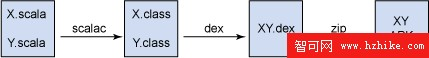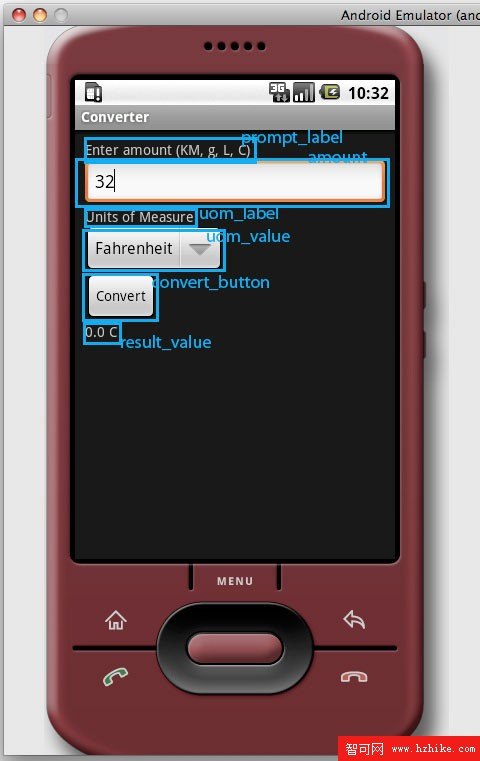﻿ 使用 Android、Scala 和 Eclipse 創建移動應用程序_開發入門Android教程網 >> Android技術 >> Android開發 >> 開發入門 >> 使用 Android、Scala 和 Eclipse 創建移動應用程序

# 使用 Android、Scala 和 Eclipse 創建移動應用程序`UnitsConverter`

```
<?xml version="1.0" encoding="utf-8"?>
<RelativeLayout XMLns:android="http://schemas.android.com/apk/res/android"
android:layout_width="fill_parent" android:layout_height="fill_parent"
>
<TextView android:id="@+id/prompt_label" android:layout_width="wrap_content"
android:layout_height="wrap_content"
android:text="@string/prompt_metric"/>
<EditText android:id="@+id/amount" android:layout_below="@id/prompt_label"
android:layout_width="fill_parent"
android:layout_height="wrap_content"/>
<TextView android:id="@+id/uom_label"
android:layout_below="@id/amount"
android:layout_width="wrap_content"
android:layout_height="wrap_content"
android:text="@string/uom"/>
<Spinner android:id="@+id/uom_value"
android:layout_below="@id/uom_label"
android:layout_width="wrap_content"
android:layout_height="wrap_content"/>
<Button android:id="@+id/convert_button"
android:layout_below="@id/uom_value"
android:layout_width="wrap_content"
android:layout_height="wrap_content"
android:text="@string/convert_button_label"/>
<TextVIEw android:id="@+id/result_value"
android:layout_below="@id/convert_button"
android:layout_width="fill_parent"
android:layout_height="fill_parent"/>
</RelativeLayout>
``````
<?XML version="1.0" encoding="utf-8"?>
<resources>
<string name="prompt_metric">Enter amount (KM, g, L, C)</string>
<string name="prompt_english">Enter amount (miles, lbs, gallons,
F)</string>
<string name="uom">Units of Measure</string>
<string name="convert_button_label">Convert</string>
<string name="app_name">Converter</string>
<string name="english_units">English</string>
<string name="metric_units">Metric</string>
</resources>
```

```
<?XML version="1.0" encoding="utf-8"?>
<resources>
<array name="english_units">
<item>Fahrenheit</item>
<item>Pounds</item>
<item>Ounces</item>
<item>Fluid Ounces</item>
<item>Gallons</item>
<item>Miles</item>
<item>Inches</item>
</array>
<array name="metric_units">
<item>Celsius</item>
<item>Kilograms</item>
<item>Grams</item>
<item>Millileters</item>
<item>Liters</item>
<item>Kilometers</item>
<item>Centimeters</item>
</array>
</resources>
```

Scala 應用程序代碼

Converter 應用程序的代碼非常簡單 — 不管用什麼語言編寫。當然，用 Java 編寫起來非常容易，但是用 Scala 編寫也同樣不復雜。首先我們看看前面見過的 UI 背後的代碼。

```
class Converter extends Activity{
import ConverterHelper._
private[this] var amountValue:EditText = null
private[this] var uom:Spinner= null
private[this] var convertButton:Button = null
private[this] var resultValue:TextView = null

override def onCreate(savedInstanceState:Bundle){
super.onCreate(savedInstanceState)
setContentView(R.layout.main)
uom = findViewById(R.id.uom_value).asInstanceOf[Spinner]
this.setUomChoice(ENGLISH)
amountValue = findViewById(R.id.amount).asInstanceOf[EditText]
convertButton = findViewById(R.id.convert_button).asInstanceOf[Button]
resultValue = findViewById(R.id.result_value).asInstanceOf[TextView]
convertButton.setOnClickListener( () => {
val unit = uom.getSelectedItem.asInstanceOf[String]
val amount = parseDouble(amountValue.getText.toString)
val result = UnitsConverter.convert(Measurement(unit,amount))
resultValue.setText(result)
})
}
true
}
setUomChoice(if (item.getItemId == 1) METRIC else ENGLISH)
true
}
private
def setUomChoice(unitOfMeasure:UnitsSystem){
if (uom == null){
uom = findViewById(R.id.uom_value).asInstanceOf[Spinner]
}
val arrayId = unitOfMeasure match {
case METRIC => R.array.metric_units
case _ => R.array.english_units
}
val units = new ArrayAdapter[String](this, R.layout.spinner_vIEw,
getResources.getStringArray(arrayId))
}
}
```

`onCreate` 中的大部分代碼類似於 Java 語言編寫的代碼。但是有幾點比較有趣。注意，我們使用 `findVIEwById` 方法（在 `Activity` 子類中定義）獲得不同 UI 元素的句柄。這個方法不是類型安全的，需要進行類型轉換（cast）。在 Scala 中，要進行類型轉換，可使用參數化方法 `asInstanceOf[T]`，其中 `T` 是要轉換的類型。這種轉換在功能上與 Java 語言中的轉換一樣。不過 Scala 有更好的語法。接下來，注意對 `setUomChoice` 的調用（稍後我們將詳細談到這個方法）。最後，注意上述代碼獲得一個在布局 XML 中創建的按鈕的句柄，並添加一個單擊事件處理程序。

android 上的函數式編程

```
object ConverterHelper{
import android.view.View.OnClickListener
implicit def funcToClicker(f:View => Unit):OnClickListener =
new OnClickListener(){ def onClick(v:View)=f.apply(v)}
implicit def funcToClicker0(f:() => Unit):OnClickListener =
new OnClickListener() { def onClick(v:VIEw)=f.apply}
}
```

```
case class Measurement(uom:String, amount:Double)

object UnitsConverter{
// constants
val lbToKg = 0.45359237D
val ozToG = 28.3495231
val fOzToMl = 29.5735296
val galToL = 3.78541178
val milesToKm = 1.609344
val inchToCm = 2.54

def convert (measure:Measurement)= measure.uom match {
case "Fahrenheit" => (5.0/9.0)*(measure.amount - 32.0) + " C"
case "Pounds" => lbToKg*measure.amount + " kg"
case "Ounces" => ozToG*measure.amount + " g"
case "Fluid Ounces" => fOzToMl*measure.amount + " mL"
case "Gallons" => galToL*measure.amount + " L"
case "Miles" => milesToKm*measure.amount + " km"
case "Inches" => inchToCm*measure.amount + " cm"
case "Celsius" => (9.0/5.0*measure.amount + 32.0) + " F"
case "Kilograms" => measure.amount/lbToKg + " lbs"
case "Grams" => measure.amount/ozToG + " oz"
case "Millileters" => measure.amount/fOzToMl + " fl. oz."
case "Liters" => measure.amount/galToL + " gallons"
case "Kilometers" => measure.amount/milesToKm + " miles"
case "Centimeters" => measure.amount/inchToCm + " inches"
case _ => ""
}
}
```

`Measurement` 是一個 case 類。這是 Scala 中的一個方便的特性。用 “case” 修飾一個類會導致這個類生成這樣一個構造函數：這個構造函數需要類的屬性，以及 `equals`、 `hashCode` 和 `toString` 的實現。它對於像 `Measurement` 這樣的數據結構類非常適合。它還為定義的屬性（在這裡就是 `uom` 和 `amount`）生成 getter 方法。也可以將那些屬性定義為 vars（可變變量），然後也會生成 setter 方法。僅僅一行 Scala 代碼可以做這麼多事情！

UI 初始化和菜單

```
sealed case class UnitsSystem()
case object ENGLISH extends UnitsSystem
case object METRIC extends UnitsSystem
```

android 平台的架構使它可以用於在 Java 虛擬機上運行的任何編程語言。我們看到了如何設置 Android 項目，使它使用 Scala 代碼。這個過程也可以延伸到其他 JVM 編程語言，例如 Groovy、JRuby 或 Fan。當可以任意使用 Scala 編程語言時，編寫 android 應用程序將變得更輕松。您仍可以使用 Eclipse 進行開發。仍然可以在 Eclipse 中用模擬器和設備進行調試。您可以繼續使用所有的工具，同時又得到一種生產率更高的編程語言。